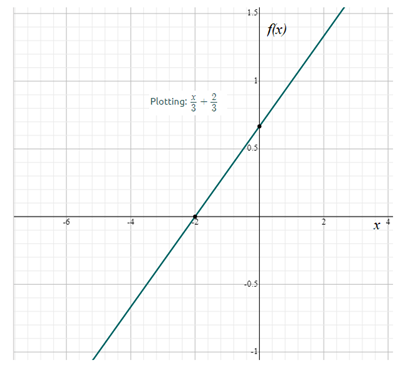# Divide the input x by 3 and add 2 3 with that gives the function f ( x ) as f ( x ) = x 3 + 2 3### Precalculus: Mathematics for Calcu...

6th Edition
Stewart + 5 others
Publisher: Cengage Learning
ISBN: 9780840068071### Precalculus: Mathematics for Calcu...

6th Edition
Stewart + 5 others
Publisher: Cengage Learning
ISBN: 9780840068071

#### Solutions

Chapter 2.1, Problem 65E
To determine

## Divide the input x by 3 and add 23 with that gives the function f(x) as f(x)=x3+23

Expert Solution

The required values of the given statement is shown in table as below:

 x 2 4 6 8 f(x) 43 2 83 103

### Explanation of Solution

Given information: Given statement is divide the input by 3 and add 23 .

Calculation:

To find some numeric values, substitute any values for x . Now substitute 2 for x , then

f(2)=23+23       =2+23        =43

Substitute 4 for x in the above equation, then

Substitute 6 for x in the above function, then

f(6)=63+23        =6+23        =83

Substitute 8 for x in the above function, then

f(8)=83+23       =8+23       =103

Thus, the required values of the given statement are shown in table as below:

 x 2 4 6 8 f(x) 43 2 83 103

Graph of the function f(x)=x3+23 as shown below:### Have a homework question?

Subscribe to bartleby learn! Ask subject matter experts 30 homework questions each month. Plus, you’ll have access to millions of step-by-step textbook answers!